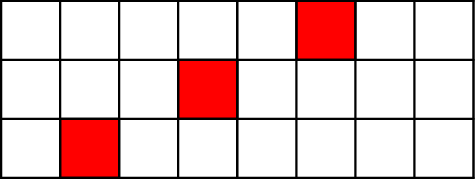# Internet Problem Solving Contest

## Problem U – Ultimate magic rectangles

Bob is busy today, so Alice has found a single-player game.

### Problem specification

In this game, Alice is given an integer s and an empty table with r = 3 rows and c columns. Alice has to fill in some nonnegative integers into the cells of the table.

Three cells are called a triplet if they lie in different rows and their centers lie on a straight line. The goal of the game is to fill the table in such a way that each triplet will have the same sum.

You are given the number of columns c and the desired sum of each triplet s. Compute the number of ways to fill the table in the desired way. Since this number may be large, compute it modulo 109 + 9.Above: one of the many triplets on a board with c = 8 columns.

### Input specification

The first line of the input file contains an integer t specifying the number of test cases. Each test case is preceded by a blank line.

Each test case consists of one line containing two space-separated integers c and s.

In the easy subproblem U1, 1 ≤ c ≤ 50 and 0 ≤ s ≤ 50.

In the hard subproblem U2, 1 ≤ c ≤ 1000 and 0 ≤ s ≤ 109.

### Output specification

For each test case, print one integer on a separate line – the number of solutions, modulo 109 + 9.

### Example

Input:

``````2

3 1

4 3``````

Output:

``````5
34``````

In the first test case there are five triplets: each column and both main diagonals of the 3 × 3 square. The sum of each triplet must be 1, which means that each triplet must contain two 0s and a 1. These are the five solutions:

``````111   000   000   101   010
000   111   000   000   000
000   000   111   010   101``````

In the second test case one of the 34 valid solutions is a 3 × 4 rectangle full of 1s.